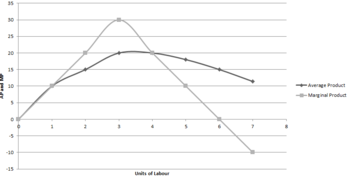Relationship between Average Product(AP) and Marginal Product(MP)Average product is per unit product of the variable factor. In other words, average product is obtained by dividing total product by the total units of variable factor used. Thus,
AP= TP/L
Where,
AP= Average Product
TP= Total Product
L= Labour

Marginal Product is the change in the total product due to change in the variable facto. In other words, marginal product is the addition to the total product by the employment of an additional unit of variable factor.
MP= TPn - TPn-1
Where,
MP= Marginal Product
n= Number of labour units
TP= Total Product

The relationship between AP and MP can be explained with the help of the following table and figure :

 Units of Labour(L) Total Product(TP) Average Product(AP) Marginal Product(MP) 0 0 0 0 1 10 10 10 2 30 15 20 3 60 20 30 4 80 20 20 5 90 18 10 6 90 15 0 7 80 11.4 -10As shown in the above table and figure, the relationship between AP and MP can be summarized as,
i. When MP > AP ,AP is rising.
ii. When MP = AP , AP is at its maximum.
iii. When MP < AP, AP is falling.
iv. MP may be positive , negative, or zero but AP is always positive.
v. When MP is rising , AP must rise but when MP is falling, AP may rise or fall.